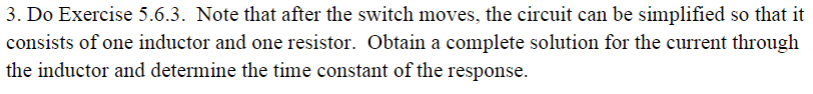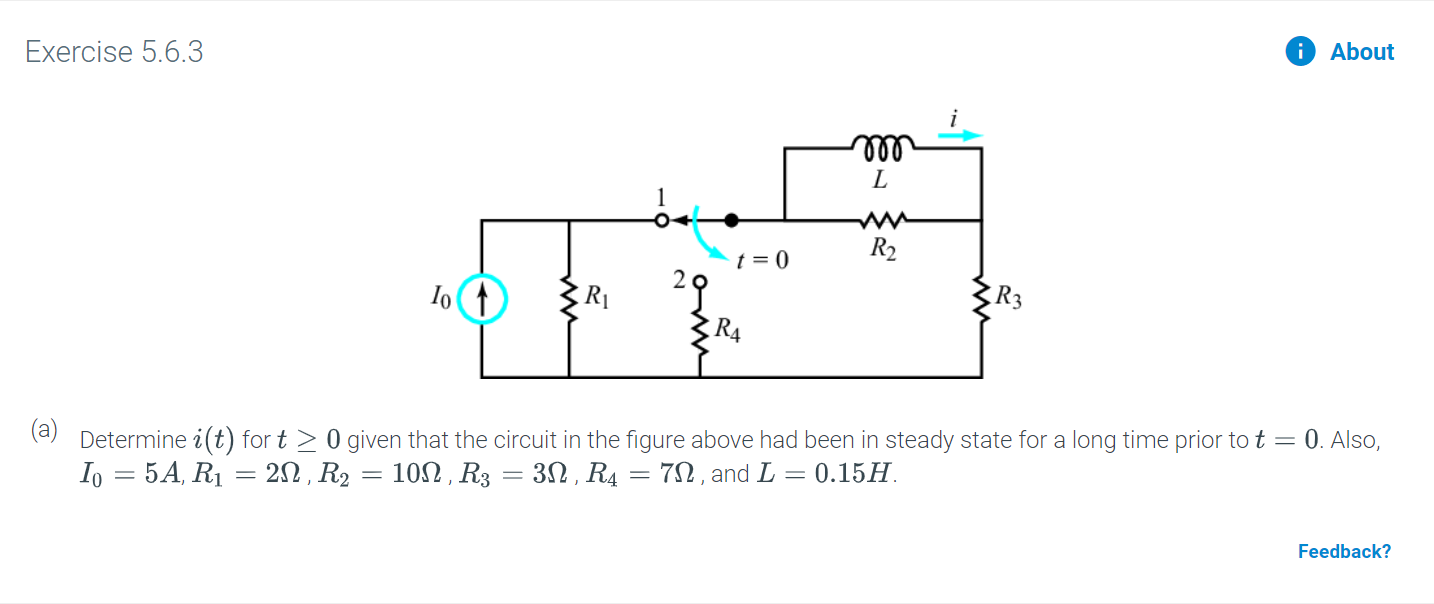# 3. Do Exercise 5.6.3. Note that after the switch moves, the circuit can be simplified so that itconsists of one inductor and one resistor. Obtain a complete solution for the current throughthe inductor and determine the time constant of the response Exercise 5.6.3AboutLR2t = 02오R4(a)Determine i(t) for t 0 given that the circuit in the figure above had been in steady state for a long time prior to tIo 5A, R1 20 R2 10, R3 = 30, R4 = 7n, and L 0.150. Also,Feedback?

Questionhelp_outlineImage Transcriptionclose3. Do Exercise 5.6.3. Note that after the switch moves, the circuit can be simplified so that it consists of one inductor and one resistor. Obtain a complete solution for the current through the inductor and determine the time constant of the response fullscreenhelp_outlineImage TranscriptioncloseExercise 5.6.3 About L R2 t = 0 2오 R4 (a) Determine i(t) for t 0 given that the circuit in the figure above had been in steady state for a long time prior to t Io 5A, R1 20 R2 10, R3 = 30, R4 = 7n, and L 0.15 0. Also, Feedback? fullscreen
check_circleExpert Solution
Step 1

Given parameters are,

Step 2

At t < 0, the switch is closed and the modified circuit is drawn as Figure 1.

Step 3

The current through the short circuit path can be calcul...

### Want to see the full answer?

See Solution

#### Want to see this answer and more?

Solutions are written by subject experts who are available 24/7. Questions are typically answered within 1 hour*

See Solution
*Response times may vary by subject and question
Tagged in

### Electrical Engineering